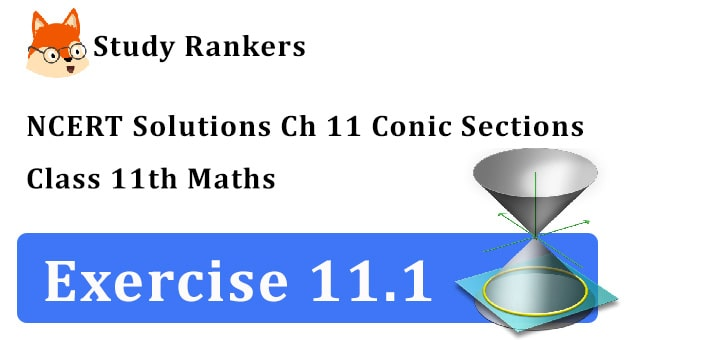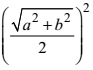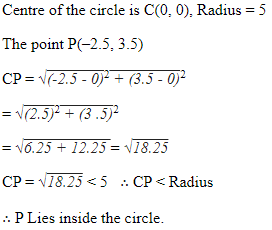## NCERT Solutions for Class 11 Maths Chapter 11 Conic Sections Exercise 11.1

NCERT Solutions of Chapter 11 Conic Sections Exercise 11.1 is provided here which will help you solving difficult questions easily and completing your homework in no time. NCERT Solutions for Class 11 Maths are prepared by Studyrankers experts that are detailed and correct so you can improve your score in the examinations.1. Find the equation of the circle with centre (0, 2) and radius 2.

Here h = 0, k = 2 and r = 2. Therefore, the required equation of the circle is
(x – 0)2 + (y – 2)2 = (2)2
or x 2 + y 2 – 4y + 4 = 4
or x 2 + y 2 – 4y = 0

2. Find the equation of the circle with centre (–2, 3) and radius 4.

Here h = –2, k = 3 and r = 4. Therefore, the required equation of the circle is
[x – (–2)]2 + (y – 3)2 = (4)2
or (x + 2)2 + (y – 3)2 = 16
or x 2 + 4x + 4 + y 2 – 6y + 9 = 16.

3. Find the equation of the circle with centre (1/2 , 1/4) and radius 1/12.

Equation of the circle is
(x – 1/2)2 + (y – 1/4)2 = 1/122 = 1/144
=> x2 + y2 – x – y/2 + 1/4 + 1/16 = 1/144
=> 36x 2 + 36y 2 – 36x – 18y + 11 = 0.

4. Find the equation of the circle with centre (1, 1) and radius √2.

Centre of circle is (1, 1), radius = √2
Equation of circle is
(x – 1)2 + (y – 1)2 = (√2)2 =2
or x2 + y2 – 2x – 2y + 2 = 2
or x2 + y– 2x – 2y = 0.

5. Find the equation of the circle with centre (–a, –b) and radius √a2 + b2

Centre of circle is (–a, –b), radius =√a2 + b2
∴ Equation of the circle is
(x + a)2 + (y + b)2 = a2 – b2
Or x+ y2 + 2xa + 2yb + a2 + b2 = a2 – b2
Or x2 + y2 + 2ax + 2by + 2b2 = 0.

6. Find the centre and radius of the circle.
(x + 5)+ (y – 3)2 = 36

Comparing the equation of the circle
(x + 5)2 + (y – 3)2 = 36
with (x – h)2 + (y – k)2 = 2
∴ –h = 5 or h = –5, k = 3, r 2 = 36, r = 6
∴ Centre of the circle is (–5, 3) and radius = 6

7. Find the centre and radius of the circle.
x2 + y2 – 4x – 8y – 45 = 0

The given equation is
x2 + y2 – 4x – 8y – 45 = 0
or (x2 – 4x) + (y2 – 5y) = 45
Now completing the squares with in the parenthesis, we get
(x 2 – 4x + 4) + (y 2 – 8y + 16) = 4 + 16 + 45
or (x – 2)2 + (y – 4)2 = 65
Therefore, the given circle has centre at (2, 4) and radius √65.

8. Find the centre and radius of the circle.
x2 + y2 – 8x + 10y – 12 = 0.

The given equation is
x2 + y2 – 8x + 10y – 12 = 0
or (x 2 – 8x) + (y 2 + 10) = 12
or (x 2 – 8x + 16) + (y 2 + 10y + 25) = 12 + 16 + 25
or (x – 4)2 + (y + 5)2 = 53
Therefore, the given circle has centre at (4, –5) and radius √53.

9. Find the centre and radius of the given circle
2x 2 + 2y 2 – x = 0.

Equation of circle is 2x 2 + 2y 2 – x = 0
=> x2 + y2 – x/2 = 0 => (x2 – x/2) + y2 = 0
=> (x2 – x/2 + 1/16) + y2 = 1/16
=> (x – 1/4)2 + y2 = 1/16
Centre is (1/4 , 0)and radius is 1.

10. Find the equation of the circle passing through the points (4, 1) and (6, 5) and whose centre is on the line 4x + y = 16.

Let the equation of the circle be
(x – h)2 + (y – k)2 = r2 … (i)
The points (4, 1) and (6, 5) lies on it
∴ (4 – h)2 + (1 – k)= r2
=> h2 + k– 8h – 2k + 17 = r2 …..(ii)
and (6 – h)2 + (5 – k)2 = r2 …..(iii)
The centre (h, k) lies on
4x + y = 16
4h + k = 16 … (iv)
Subtracting (iii) from (ii),
∴ 4h + 8k – 44 = 0 Þh + 2k = 11 … (v)
Multiplying (v) by 4, 4h + 8k = 44
Subtracting eqn (iv) from it
7k = 44 – 16 = 28 ∴ k = 4
From (v) h + 8 = 11 ∴ h = 3
Putting h = 3, k = 4 in (ii)
9 + 16 – 24 – 8 + 17 = r2
=> 42 – 32 = r 2
∴ r 2 = 10
∴ Equation of the circle is
(x – 3)2 + (y – 4)2 = 10
=> x 2 + y 2 – 6x – 8y + 15 = 0

11. Find the equation of the circle passing through the points (2, 3) and (–1, 1) and whose centre is on the line x – 3y – 11 = 0.

Let the equation of the circle be
(x – h) 2 + (y – k) 2 = r 2 … (i)
Since, the points (2, 3) and (–1, 1) lies on it.
∴ (2 – h) 2 + (3 – k) 2 = r 2
h 2 + k 2 – 4h – 6k + 13 = r 2 … (ii)
Centre (h, k) lies on x – 3y – 11 = 0
h – 3k – 11 = 0 … (iii)
Subtracting (ii) from (i) 6h + 4 k – 11 = 0 … (iv)
Multiply eqn (iii) by 6 6h – 18 k – 66 = 0 … (v)
Subtracting (v) from (iv) 22k + 55 = 0
∴ k = -(55/22) = -(5/2)
from (iii) h = 3k + 11 = -(15/2) + 11 = 7/2
Put the value of h and k in (2 – h) 2 + (3 – k) 2 = r2
(2 – 7/2)2 + (3 + 5/2)2 = r2
=> r2 = 9/4 + 121/4 130/4 = 65/2
∴ Equation of the circle
(x – 7/2)+ (y + 5/2)2 = 65/2
=> x2 + y2 – 7x + 5y + 49/4 + 25/4 – 65/2 = 0
=> x2 + y2 – 7x + 5y – 14 = 0

12. Find the equation of the circle with radius 5 whose centre lies on x-axis and passes through the point (2, 3).

Let the equation of the circle be
(x – h)2 + (y – k)2 = r2 … (i)
r = 5 ∴ r 2 = 25
Centre lies on x -axis is k = 0
Equation (i) becomes (x – h) 2 + y 2 = 25 (2, 3) lies on it
∴ (2 – h) 2 + 9 = 25 => (2 – h) 2 = 16,
∴ 2 – h = ±4 => h = –2, 6
When h = –2, equation of circle
(x + 2)2 + y 2 = 25
=> x2 + y2 + 4x – 21 = 0
When h = 6, (x – 6)2 + y 2 = 25,
2 + y 2 – 12x + 11 = 0
Thus, required circles are x 2 + y 2 + 4x – 21 = 0
and x2 + y 2 – 12x + 11 = 0

13. Find the equation of the circle passing through (0, 0) and making intercepts a and b on the coordinate axes.

a, b are the intercepts made by the circle on the co-ordinate axes at A and B, C the mid point of AB is the centre of the circle
∴ centre (a/2 , b/2);
radius = OC ==∴ Equation of the circle is
(x – a/2)2 + (y – b/2)2 =x2 + y2 – ax – by + a2/4 + b2/4 = (a2 + b2)4
=> x2 + y2 – ax – by = 0

14. Find the equation of a circle with centre (2, 2) and passes through the point (4, 5).